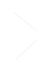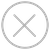TensorSpace.js
Getting StartBasic ConceptsTensorSpace ConverterModel PreprocessingModelsLayersMerge FunctionPredict
'Predict' is the way to gather the computed data from the loaded pre-trained models (e.g. TensorFlow, Keras, TensorFlow.js models). All outputs from intermediate layers are required and delivered to the corresponding visualization components.
The functionality of 'Predict' is realized by .predict( inputData, callback ) method of the constructed model object.
After we Load a ML model properly we can simply call the method as:
``model.predict( predictData, callback );``
predictData
predictData is actually a list. It could be one or more lists of numbers.
filter_center_focus For Sequential() models
In most cases, we can configure the predictData like:
``predictData = [ 1, 2, 3, 4, 5 ];``
The predictData is a single list of numbers which is the input image data we want to used for prediction.
If the model accepts multiple inputs, we ned to configure the predictData like:
``````let data1 = [ 1, 2, 3, 4, 5 ];
let data2 = [ 1, 2, 3, 4, 5 ];
predictData = [ data1, data2 ];``````
The predictData contains some lists of numbers.
filter_center_focus For Model() models
For example, we can configure the predictData like:
``````let data1 = [ 1, 2, 3, 4, 5 ];
let data2 = [ 1, 2, 3, 4, 5 ];
predictData = [ data1, data2 ];``````
The predictData contains some lists of numbers. Depends on the model structure, we may add one or more list in the predictData.
callback
callback function is fired when the prediction process complete. It passes the final inferences as the parameter in the callback function.
``````function predictCallback( finalResult ) {
console.log( finalResult );
}``````
All the following steps are triggered by calling the .predict() method: the model collects all necessary inferences data from each layer, then the collected data are used to render the corresponding 3D components.
wb_sunnyNote:
• filter_center_focus Please check the configurations before you apply the multiple inputs.
• filter_center_focus See Load for more details.